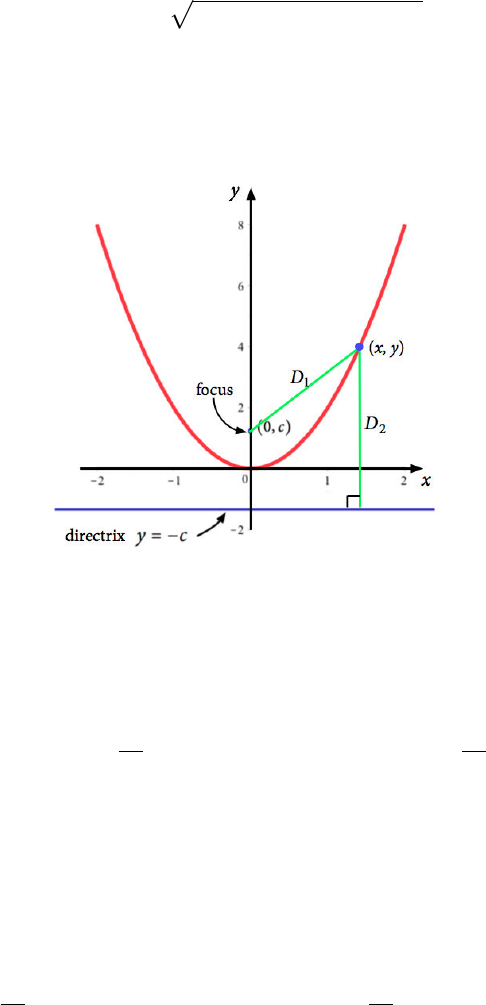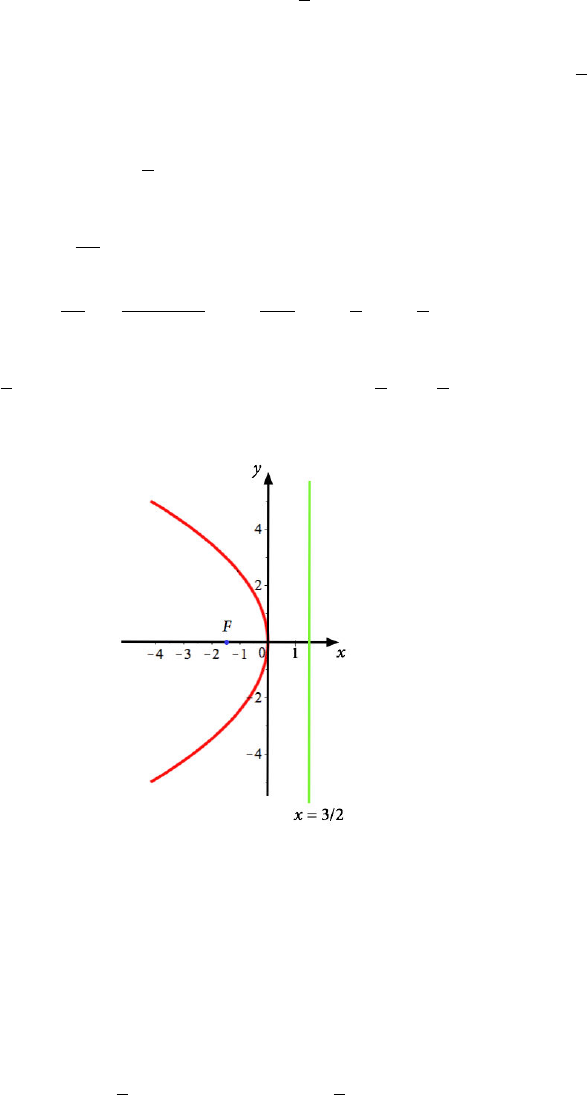Study Guides (400,000)
US (230,000)
NCSU (600)
MA (50)
MA 141 (40)
Final

# MA 141 Lecture 2: Conic SectionsExam

Department
Mathematics
Course Code
MA 141
Professor
Kevin Flores
Study Guide
Final

This preview shows pages 1-2. to view the full 6 pages of the document.MA 141 Chapter 0
Section 0.2: Conic Sections
Distance Formula: (derived from Pythagorean Theorem) Given two points P= (x1, y1)
and Q= (x2, y2)in R2, the distance between them is
d(P, Q) = p(x1x2)2+ (y1y2)2.
Def: Aparabola is the set of points in the xy-plane that are equidistant from a ﬁxed point,
the focus, and a ﬁxed line, the directrix. The vertex is the point of the parabola that
minimizes the distance to the focus and directrix.
D1=D2
The standard equation of the vertical parabola is
yk=a(xh)2.
In this case, the focus is h, k +1
4aand the directrix is y=k1
4a.
If a > 0, the parabola opens upward and the vertex is the lowest point of the graph. If a < 0,
the parabola opens downward and the vertex is the highest point of the graph.
Similarly, we can have a parabola opening left or right. The standard equation in this
case is
xh=a(yk)2.
The focus is at h+1
4a, kand the directrix is x=h1
4a.
If a > 0, the parabola opens to the right and the vertex is the left-most point. If a < 0, the
parabola opens left and the vertex is the right-most point.
1

Only pages 1-2 are available for preview. Some parts have been intentionally blurred.Ex: Find the focus, vertex, and directrix of x=1
6y2and then sketch the parabola.
This parabola is of the second type, opening either left or right. Since a=1
6is negative,
it opens to the left.
This can be rewritten as x0 = 1
6(y0)2, so the vertex is at (0,0).
The focus is then given by 1
4a,0.
1
4a=1
4(1/6) =1
4/6=6
4=3
2
Then the focus is 3
2,0and the directrix is x=3
2=3
2.
=
Note that if the parabola is not in the given form above, we can complete the square to
convert to the standard equation.
Recall: Given a quadratic y=x2+ 8x+ 3, we complete the square as follows.
y=x2+ 8x+ 3
y+8
22
= x2+ 8x+8
22!+ 3
y+ 16 = (x2+ 8x+ 16) + 3
y+ 13 = (x+ 4)2
Then the standard form of the parabola is y+ 13 = (x+ 4)2.